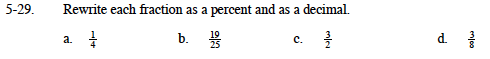### Home > MC1 > Chapter 5 > Lesson 5.1.3 > Problem5-29

5-29.0.25, 25%, or one-quarter

Can you find a way to rewrite the fraction so that the denominator is 100?

1.5

$\text{If }\frac{1}{8}\text{ is equal to }12.5\%,\text{ what percent is }\frac{3}{8}?$